open User's Guide (in this window) open Applet Page (in new window)

The Advection Upstream Splitting Method (AUSM) is a method similar in principle to the Van Leer flux splitting already discussed.  Here too, the flux is split into two separate components so that each one may be properly upwind stenciled.  Liou and Steffen (1993) are the original authors of the technique, which has been improved and altered several time since both by them and by others.  The original scheme is utilized in Gryphon, as it offers good accuracy and speed for the cases studied.  In this case, the idea is that the flux term can be divided into two pieces -- the convective flux and the pressure flux, shown in eqn. (55).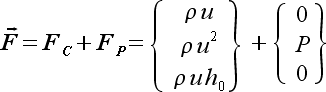(55)
The convective flux is carried by the flow, i.e. through the entropy wave.  It can be defined completely in the upwind direction by using the properties of the convective (entropy) wave.  This will be discussed just below.  The pressure term of the flux is the term that is carried by the entropy waves, which allow for travel in a direction against the flow in a subsonic situation.  This term is to be separated into two relative contributions.  First, the convective is shown in eqn. (56).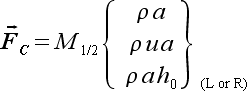(56)
The special Mach number term that has been pulled out of the convective flux term is the "convective" Mach number.  The convective Mach number is a measure of the effective convective potential of the flow, based on the strength of the entropy eigenvalue.  The convective Mach number can be defined as shown in eqn. (57).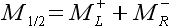(57)
The definition for the left and right Mach numbers were taken from the Van Leer method, as in eqn. (58).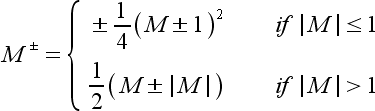(58)
This equation shows that the convective Mach will simply reduce to the full signed Mach number for any supersonic flow, while it employs Van Leer's Mach number splitting for subsonic flows.  The convective Mach number definition is one of the key variations between the successive AUSM enhancements that can be found in the literature.
The "L or R" in the remaining flux term is a switch that corresponds to the sign of the convective Mach number.  It is defined as follows in eqn. (59).(59)
In this way, the convective flux is defined upwind based on the convection of the flow.
The pressure term is treated separately.  It is defined so that communication in both directions may be admitted.  Equation (60) shows the calculation of the pressure.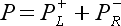(60)
The left and right pressure terms are defined by using second order polynomial functions of the acoustic speeds.  Liou and Steffen (1993) also give a slightly simpler first order polynomial splitting, but the second order scheme is used here.  These are given by eqn. (61).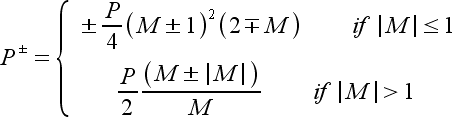(61)
Again, the pressure term becomes the full, signed, upwind pressure for any supersonic flow.  For subsonic flow, the pressure correctly shows characteristics for both upwind and downwind waves.
The flux at any cell boundary then becomes defined by eqn. (62), with supplementary definitions given in eqns. (58) and (61).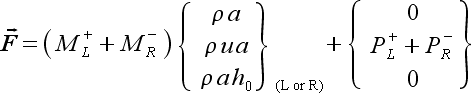(62)
This defines the flux terms necessary to evaluate the explicit residual.  For implicit schemes, the Jacobian of the flux must be computed.  Like in the previous sections, the derivative of a flux scalar with respect to a conservative variable scalar can be described as a differential using the chain rule for the left and right conservative variable states.  Since the left and right pressure and convective Mach number values shown in eqn. (62) are only functions of the corresponding one-sided state.  This feature simplifies the derivative a little bit.  Equations (63) and (64) give the left and right derivatives for any term in the Jacobian matrix.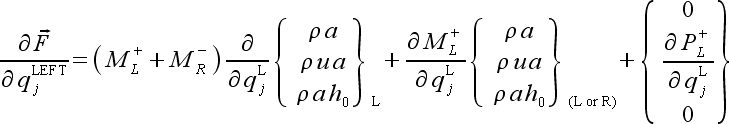(63)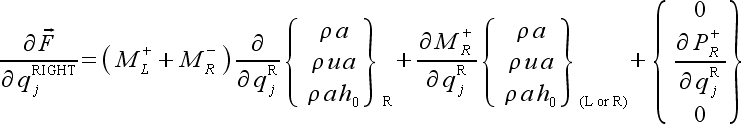(64)
The first term in each derivative can be zero if the switch does not indicate that respective direction, i.e. if the convective Mach number is positive, the convective flux willl be "LEFT" biased and the derivative of the flux with respect to the right state will be zero by definition.  Thus, in this case, the first term of eqn. (64) will be zero.  Note, however, that the second term will be non-zero since this is the actual term instead of a derivative.  For supersonic flow, the second and third terms will be zero for the downwind side of the flow.  The actual derivatives with respect to the face conservative variables for the pressure, Mach number, and flux terms are relatively straight forward by employing the chain rule.